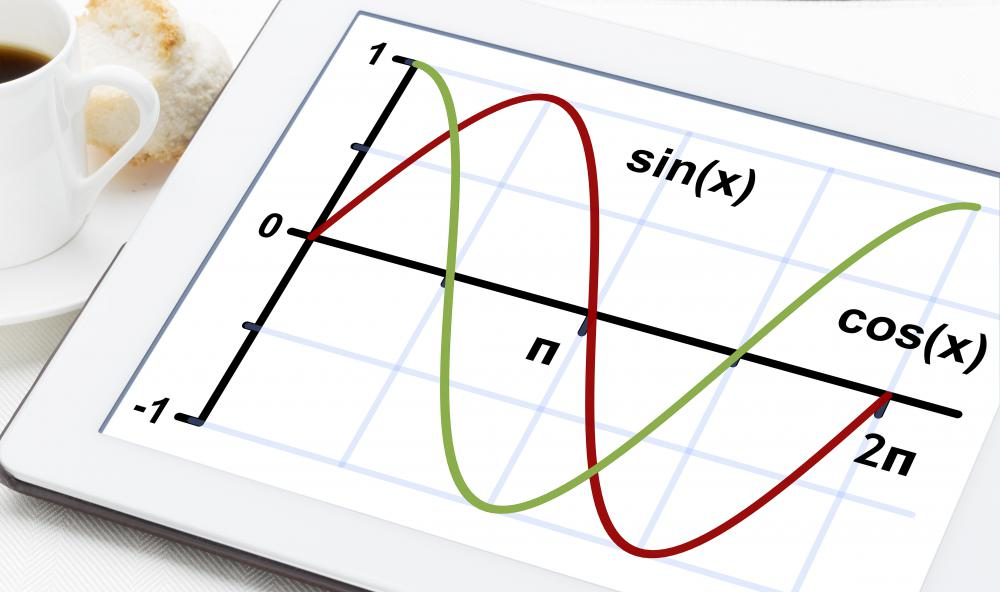# What is a Sine Graph?

Brendan McGuigan

A sine graph is a graph displaying the function of y = sin x. A sine graph has a function which may also be described as a sinusoidal wave. It repeats itself as it moves along the x-axis, and the cycle taken for a repetition is known as the period of the sine graph. A number of different analyses can be done on the period and amplitude of a sine graph, and there are many interesting results that can be gleaned from this crucial function.A sine graph is a graph displaying the function of y = sin x.

Sine itself is a measurement given to an angle, representing the ratio between the opposite of the far side to the length of the hypotenuse. It can be contrasted with the cosine, which represents the ratio between the adjacent side and the hypotenuse, and the tangent, which represents the ratio between the opposite side and the adjacent side. Each function also has a reciprocal, for example the cosecant, the reciprocal of sine, which represents the ratio between the hypotenuse and the opposite side.

The best way to understand a sine graph is to look at a visual representation of a unit circle, which demonstrates where various important values of sine fall on the different angles radiating out from a single circle. It makes it very evident when sine has a value of 0, which appear on the four points radiating out from a cross in the middle of the circle, equal to 0,1 or 1,0 or 0,-1 or -1,0. This allows us to see that the period of a sine graph is equal to 2π, with each additional period just being another loop around the circle.

On a sine graph, this can be seen as a sinusoidal wave which curves up towards a 1 value, then crosses back down below the 0 mark to -1, then turns upwards again to repeat the process. It makes a traverse from the trough to the peak every iteration of π, and returns to its previous position after 2π. The trough on the Cartesian plane appears at, for example, -π/2 and 3π/2, while the peak appears at -3π/2, π/2. A cosine graph looks very similar to a sine graph, but its peak would appear at, for example, -2π, 0, and 2π.

One can see examples of a sine wave almost everywhere, from pure mathematics to physics, to music, to electrical engineering. The sine wave is unique in that it keeps its same wave shape when another sine wave is added to it, so long as the second wave has the same frequency and phase. Lots of basic physics thought experiments can be demonstrated with a pure sine wave, from a simple pure tone to how a spring oscillates if it is completely undampened by things like friction.

In sound, a tone that would appear as a sine graph is heard by humans as a pure note. For example, steady whistle would usually make a sine wave if observed in sound recording software. The sound made by a tuning fork is another good example of a relatively pure sine wave.

## You might also Like